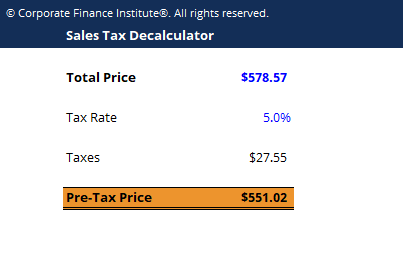# Sales Tax Decalculator Template

## Sales Tax Decalculator Template

This sales tax decalculator template will help you calculate the pre-tax price of a good or service when the total price and tax rate are known.

This is what the sales tax decalculator looks like:### Pre-tax price formula

Here is the formula for de-calculating the tax, or determining the pre-tax price of the good/service.

Pre-Tax Price  =  TP – [(TP / (1 + r) x r]

Where:

TP = Total Price

r = Tax rate

### How the decalculator works

The Excel de-calculator works by using a formula that takes the following steps:

Step 1: take the total price and divide it by one plus the tax rate

Step 2: multiply the result from step one by the tax rate to get the dollars of tax

Step 3: subtract the dollars of tax from step 2 from the total price

### More Free Templates

For more resources, check out our business templates library to download numerous free Excel modeling, PowerPoint presentation and Word document templates.

• Excel Modeling Templates
• PowerPoint Presentation Templates
• Transaction Document Templates

### Financial Analyst Certification

Become a certified Financial Modeling and Valuation Analyst (FMVA)® by completing CFI’s online financial modeling classes and training program!